Question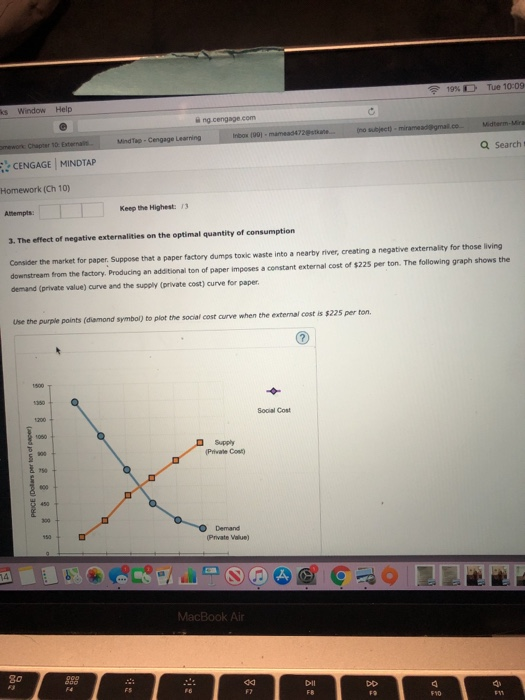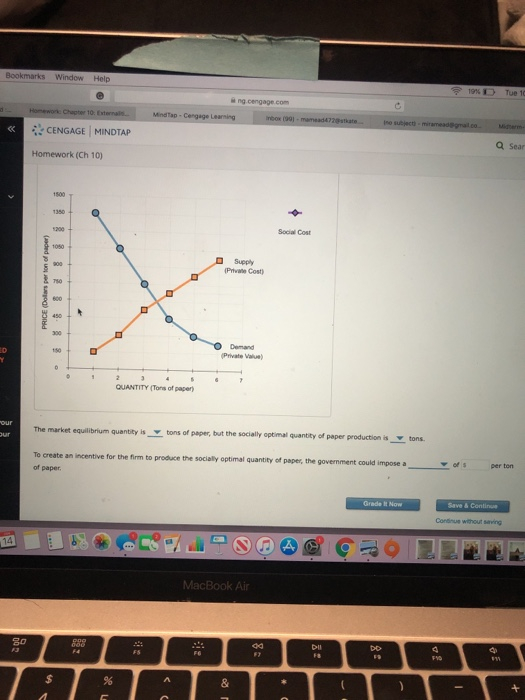The external cost is \$ 225 per ton thus the social cost would be less than the equilibrium quantity.

Q Price Private + External cost = Social Cost
1 1350 150 + 225 = 375
2 1050 300 + 225 = 525
3 750 525 + 225 = 750
4 450 675 + 225 = 900
5 300 825 + 225 = 1050
6 225 975 + 225 = 1200

The market equilibrium quantity is 3.5 tons of paper. Socially efficient paper production = 3 tons of paper.

To create an incentive for the firm to produce the socially optimal quantity of paper, the government could impose a tax of \$ 225 paper per ton.

I am really sorry I don't have access to the graphing tool thus I have constructed it manually please accept it it would be great help to me.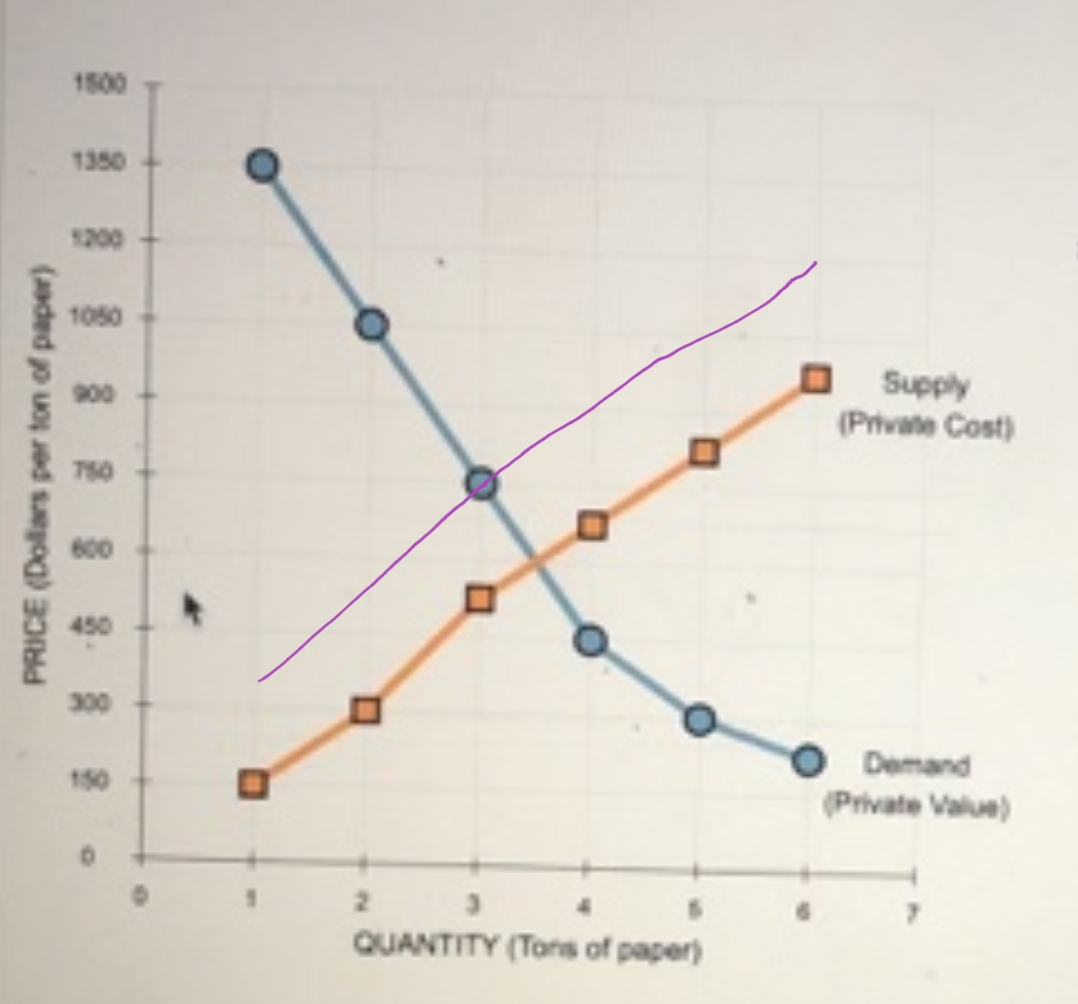#### Earn Coins

Coins can be redeemed for fabulous gifts.

Similar Homework Help Questions
• ### park confer Homework (Ch 10) 2. The effect of negative externalities on the optimal quantity of...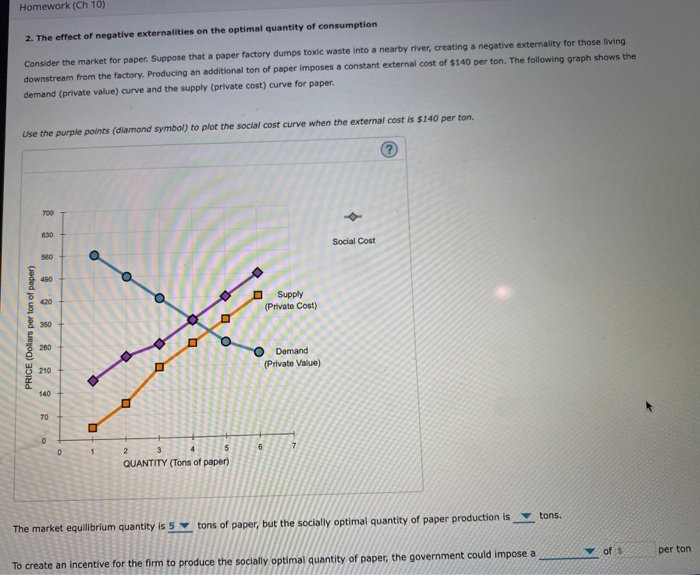park confer Homework (Ch 10) 2. The effect of negative externalities on the optimal quantity of consumption Consider the market for paper. Suppose that a paper factory dumps toxic waste into a nearby river, creating a negative externality for those living downstream from the factory Producing an additional ton of paper imposes a constant external cost of \$140 per ton. The following graph shows the demand (private value) curve and the supply (private cost) curve for paper. Use the purple...

• ### 3. The effect of negative externalities on the optimal quantity of consumption Consider the market for...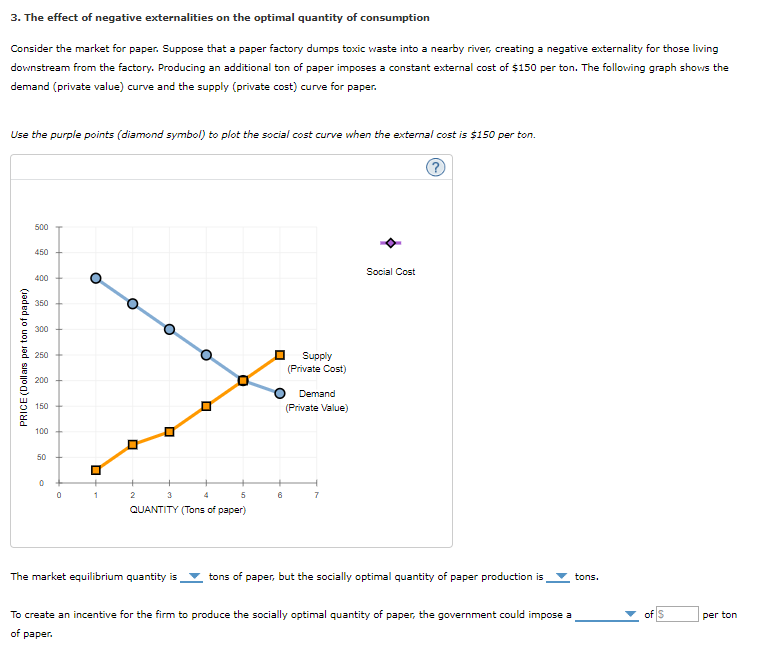3. The effect of negative externalities on the optimal quantity of consumption Consider the market for paper. Suppose that a paper factory dumps toxic waste into a nearby river, creating a negative externality for those living downstream from the factory. Producing an additional ton of paper imposes a constant external cost of \$150 per ton. The following graph shows the demand (private value) curve and the supply (private cost) curve for paper. Use the purple points (diamond symbol) to plot...

• ### PRICE (Dollars per ton of paper) Consider the market for paper. Suppose that a paper factory...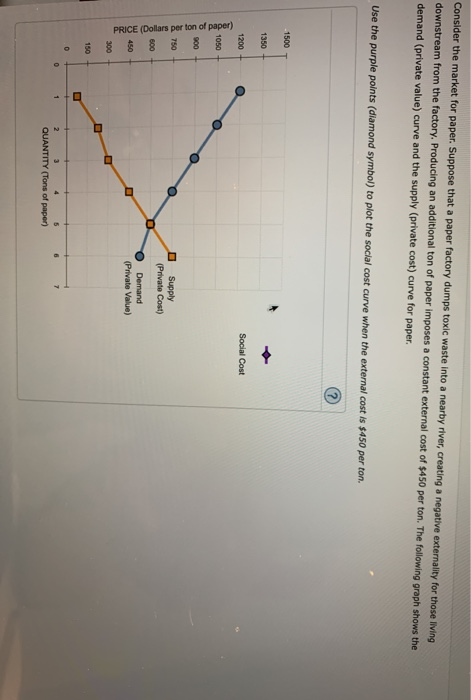PRICE (Dollars per ton of paper) Consider the market for paper. Suppose that a paper factory dumps toxic waste into a nearby river, creating a negative externality for those living downstream from the factory. Producing an additional ton of paper imposes a constant external cost of \$450 per ton. The following graph shows the demand (private value) curve and the supply (private cost) curve for paper. Use the purple points (diamond symbol) to plot the social cost curve when the...

• ### Homework (Ch 10) 3. The effect of negative externalities on the optimal quantity of consumption Consider...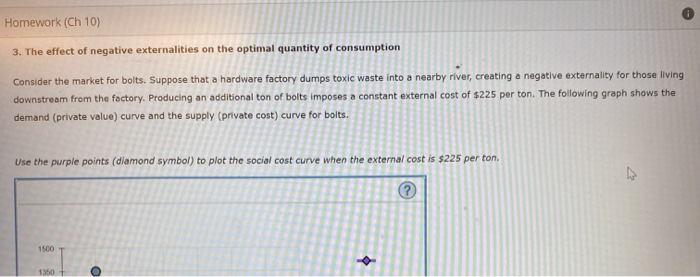Homework (Ch 10) 3. The effect of negative externalities on the optimal quantity of consumption Consider the market for bolts. Suppose that a hardware factory dumps toxic waste into a nearby river, creating a negative externality for those living downstream from the factory. Producing an additional ton of bolts imposes a constant external cost of \$225 per ton. The following graph shows the demand (private value) curve and the supply (private cost) curve for bolts. Use the purple points (diamond...

• ### Homework (Ch 10) 3. The effect of negative externalities on the optimal quantity of consumption Consider the market...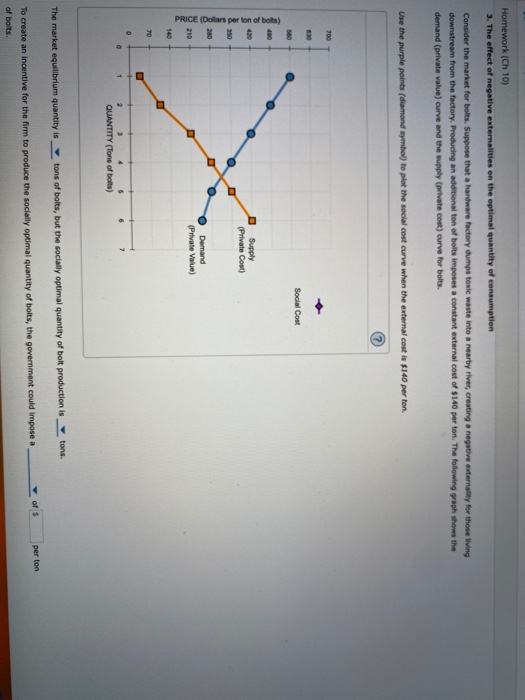Homework (Ch 10) 3. The effect of negative externalities on the optimal quantity of consumption Consider the market for bolts. Suppose that a hardware factory dumps toxic waste into a nearby river, creating a negative externality for those living downstream from the factory Producing an additional ton of bolts imposes a constant external cost of \$140 per ton. The following graph shows the demand (private value) curve and the supply (private cost) curve for bolts. Use the purple points (diamond...

• ### 3. The effect of negative externalities on the optimal quantityof consumption Consider the market for paper....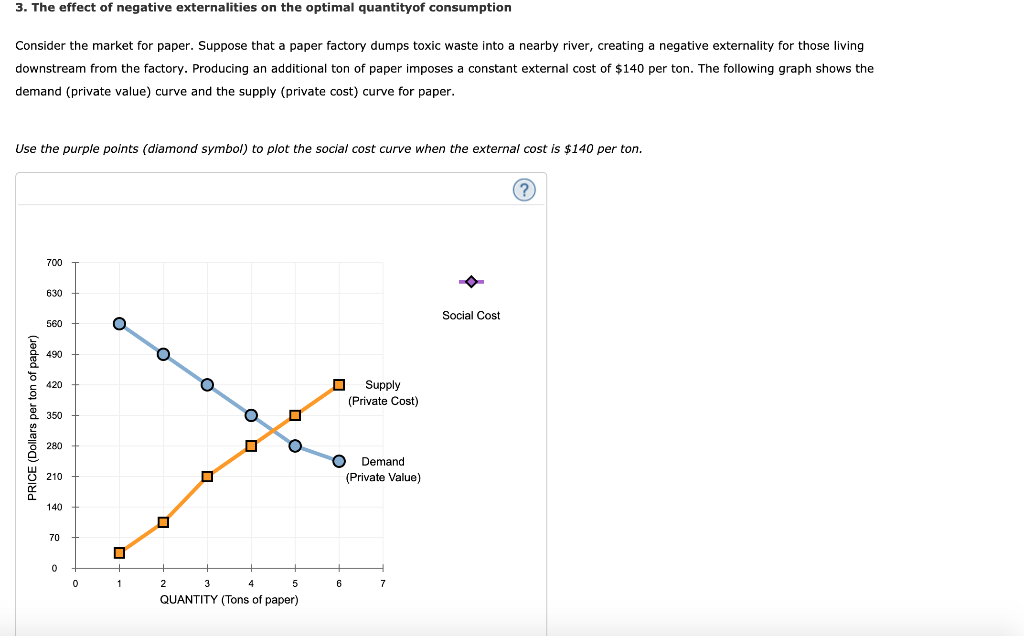3. The effect of negative externalities on the optimal quantityof consumption Consider the market for paper. Suppose that a paper factory dumps toxic waste into a nearby river, creating a negative externality for those living downstream from the factory. Producing an additional ton of paper imposes a constant external cost of \$140 per ton. The following graph shows the demand (private value) curve and the supply (private cost) curve for paper. Use the purple points (diamond symbol) to plot the...

• ### Consider the market for paper. Suppose that a paper factory dumps toxic waste into a nearby...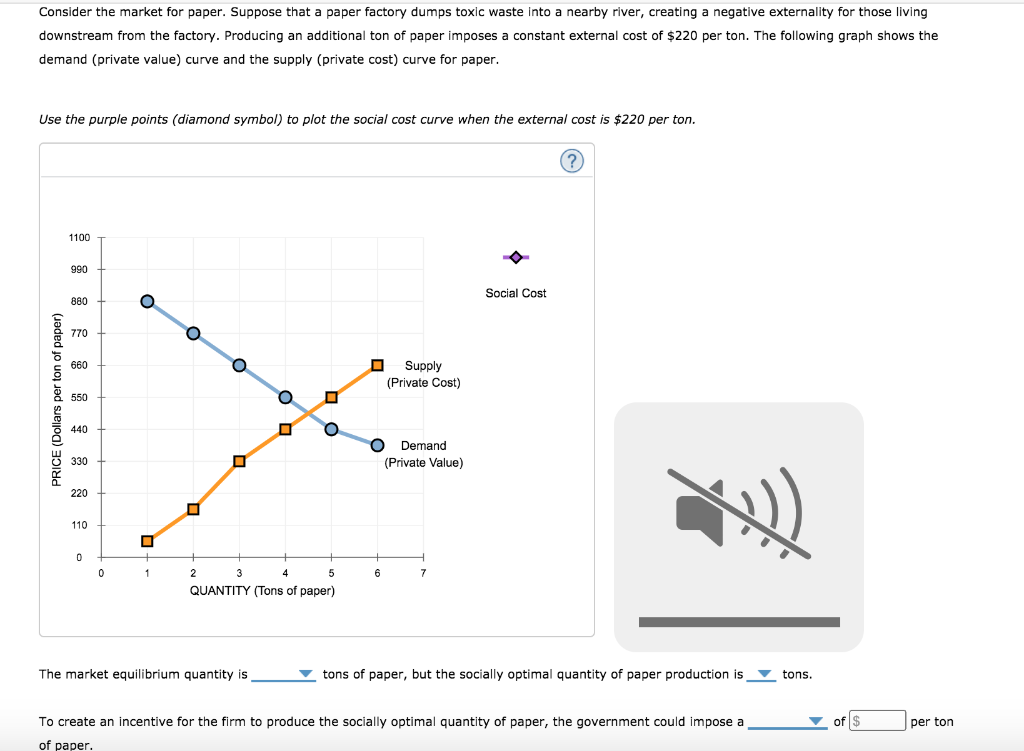Consider the market for paper. Suppose that a paper factory dumps toxic waste into a nearby river, creating a negative externality for those living downstream from the factory. Producing an additional ton of paper imposes a constant external cost of \$220 per ton. The following graph shows the demand (private value) curve and the supply (private cost) curve for paper. Use the purple points (diamond symbol) to plot the social cost curve when the external cost is \$220 per ton....

• ### Electric form is better. Thank you 3. The effect of negative externalities on the optimal quantity...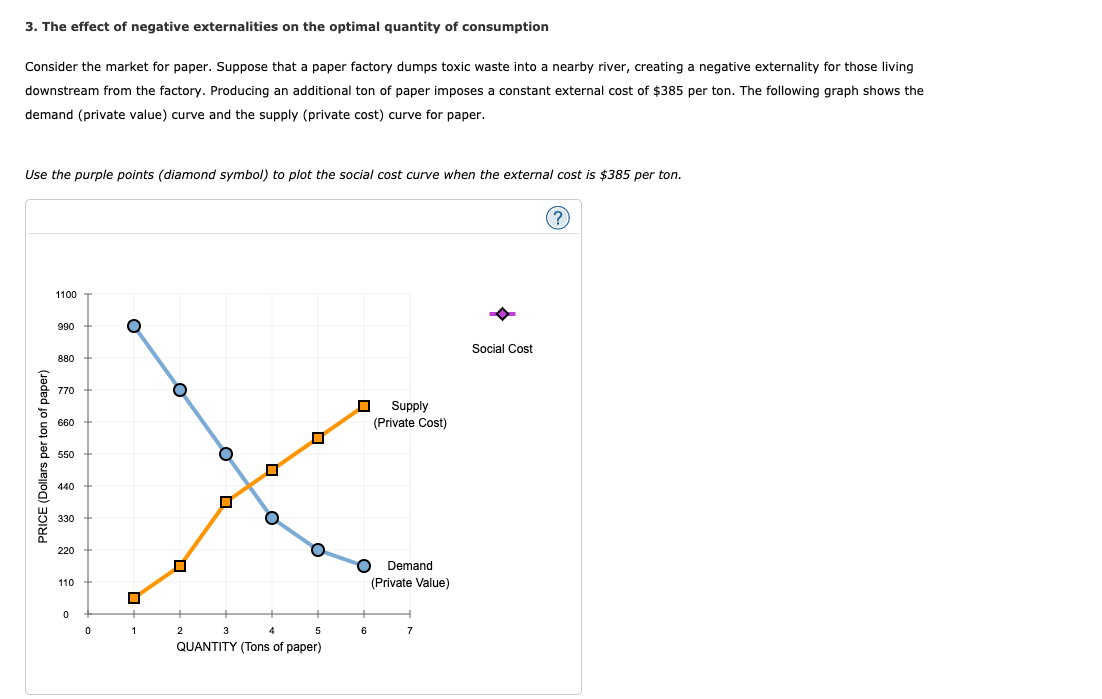Electric form is better. Thank you 3. The effect of negative externalities on the optimal quantity of consumption Consider the market for paper. Suppose that a paper factory dumps toxic waste into a nearby river, creating a negative externality for those living downstream from the factory. Producing an additional ton of paper imposes a constant external cost of \$385 per ton. The following graph shows the demand (private value) curve and the supply (private cost) curve for paper. Use the...

• ### 3. The effect of negative externalities on the optimal quantity of consumption Consider the market for...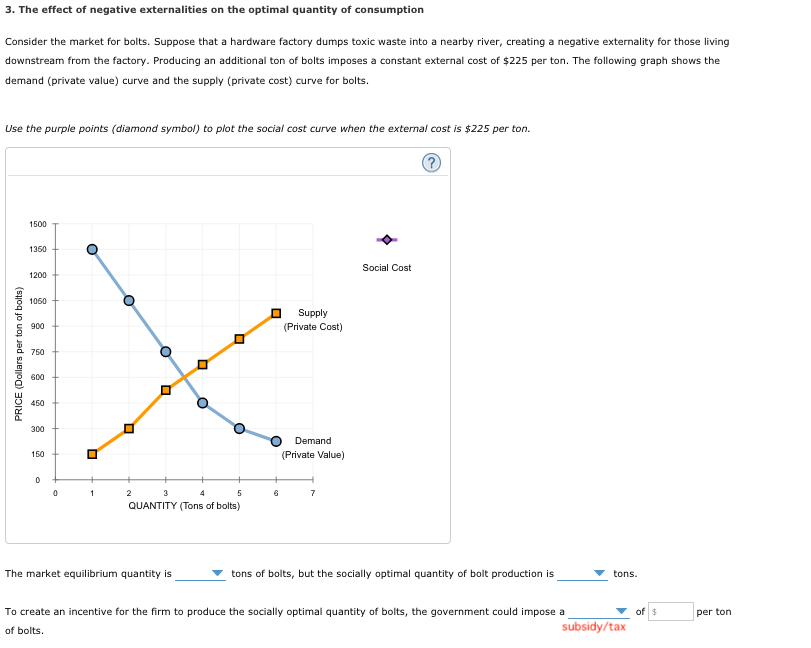3. The effect of negative externalities on the optimal quantity of consumption Consider the market for bolts. Suppose that a hardware factory dumps toxic waste into a nearby river, creating a negative externality for those living downstream from the factory. Producing an additional ton of bolts imposes a constant external cost of \$225 per ton. The following graph shows the demand (private value) curve and the supply (private cost) curve for bolts Use the purple points (diamond symbol) to plot...

• ### The effect of negative externalities on the optimal quantityof consumption Consider the market for paper. Suppose that...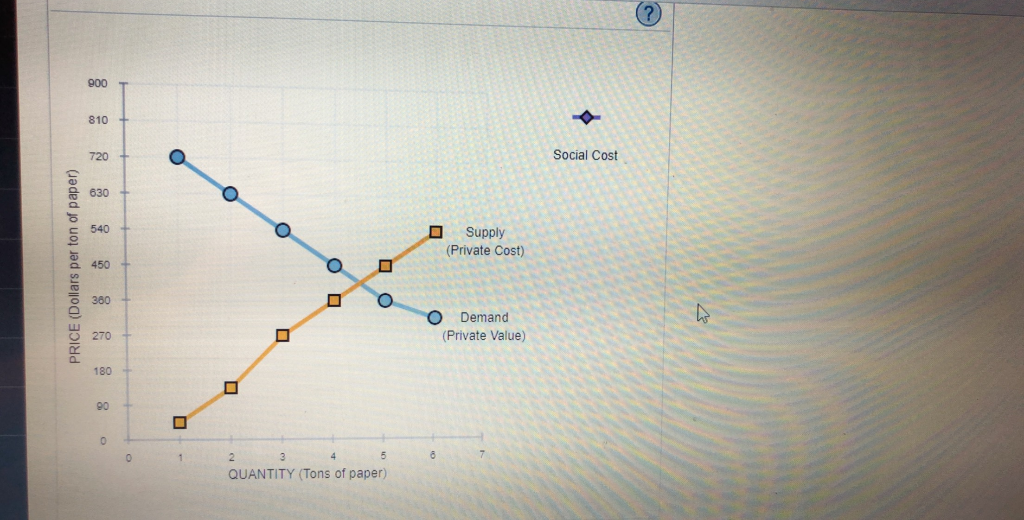The effect of negative externalities on the optimal quantityof consumptionConsider the market for paper. Suppose that a paper factory dumps toxic waste into a nearby river, creating a negative externality for those living downstream from the factory. Producing an additional ton of paper imposes a constant external cost of \$180 per ton. The following graph shows the demand (private value) curve and the supply (private cost) curve for paper.Use the purple points (diamond symbol) to plot the social cost curve...